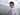# 4. 寻找两个有序数组的中位数

October 27, 2019

https://leetcode-cn.com/problems/median-of-two-sorted-arrays/

## O((m + n)/2) 解法：

``````func findMedianSortedArrays(nums1 []int, nums2 []int) float64 {
totalLen := len(nums1) + len(nums2)
midIndex := (totalLen - 1) / 2
remainder := (totalLen - 1) % 2

lastIndex := midIndex
if remainder > 0 {
lastIndex += 1
}

var i, j int
var res []int
for len(res) <= lastIndex {
if i >= len(nums1) {
res = append(res, nums2[j])
j++
continue
} else if j >= len(nums2) {
res = append(res, nums1[i])
i++
continue
}

num1 := nums1[i]
num2 := nums2[j]
if num1 > num2 {
res = append(res, num2)
j++
} else {
res = append(res, num1)
i++
}
}

if remainder == 0 {
return float64(res[len(res) - 1])
} else {
return float64(res[len(res) - 1] + res[len(res) - 2]) / 2
}
}``````

## O(log((MIN(m, n))) 解法：

``````func findMedianSortedArrays(nums1 []int, nums2 []int) float64 {
if len(nums1) > len(nums2) {
return findMedianSortedArrays(nums2, nums1)
}
x := len(nums1)
y := len(nums2)
totalLen := x + y
// k < y && k >= x; if x == y then k == x == y
k := totalLen / 2
isEven := totalLen % 2 == 0

// nums1 = [] nums2 = [*]
if k == 0 {
return float64(nums2)
}

c1 := len(nums1) / 2
c2 := k - c1

// 二分
var lMax,rMin int
lC1 := x
fC1 := 0
for c1 >= 0 && c1 <= x {
if c1 == 0 {
lMax = nums2[c2 - 1]
if c2 == len(nums2) {
rMin = nums1
} else {
rMin = nums2[c2]
if len(nums1) > 0 {
rMin = getMin(rMin, nums1)
}
}
if lMax > rMin {
lMax = 0
rMin = 0
c1++
c2 = k - c1
continue
}
break
} else if c1 == x {
if c2 == 0 {
lMax = nums1[c1 - 1]
rMin = nums2
} else {
lMax = getMax(nums1[c1 - 1], nums2[c2 - 1])
rMin = nums2[c2]
}
break
}

l1 := nums1[c1 - 1]
l2 := nums2[c2 - 1]
r1 := nums1[c1]
r2 := nums2[c2]
if l1 <= r2 && l2 <= r1 {
lMax = getMax(l1, l2)
rMin = getMin(r1, r2)
break
} else if l1 > r2 {
lC1 = c1 - 1
c1 = (lC1 + fC1) / 2
} else if l2 > r1 {
fC1 = c1 + 1
c1 = (fC1 + lC1) / 2
}
c2 = k - c1
}

// 奇数列
if !isEven {
lMax = rMin
}
return float64(lMax + rMin) / 2.0
}

func getMin(n1, n2 int) int {
if n1 > n2 {
return n2
}
return n1
}

func getMax(n1, n2 int) int {
if n1 > n2 {
return n1
}
return n2
}``````